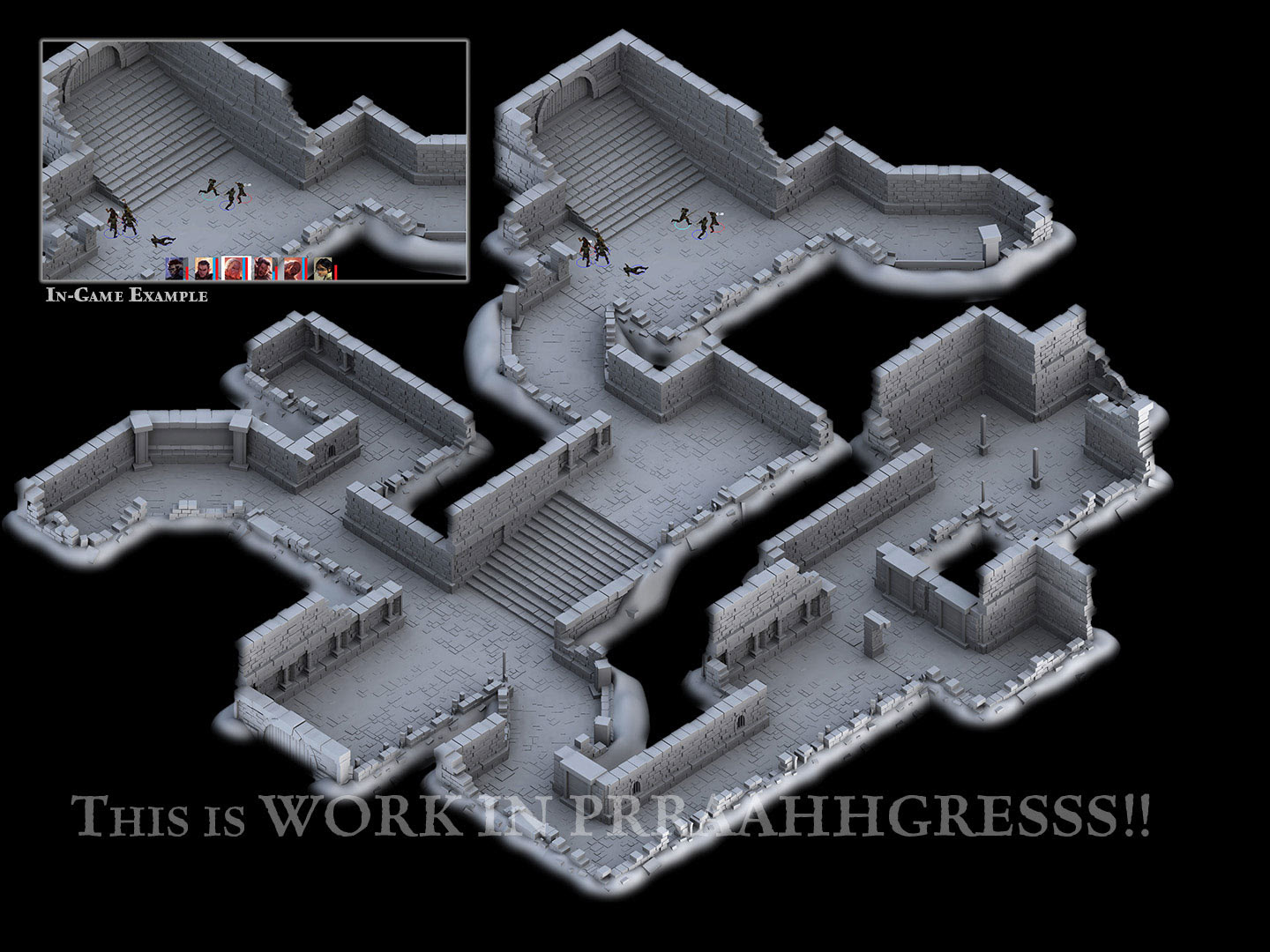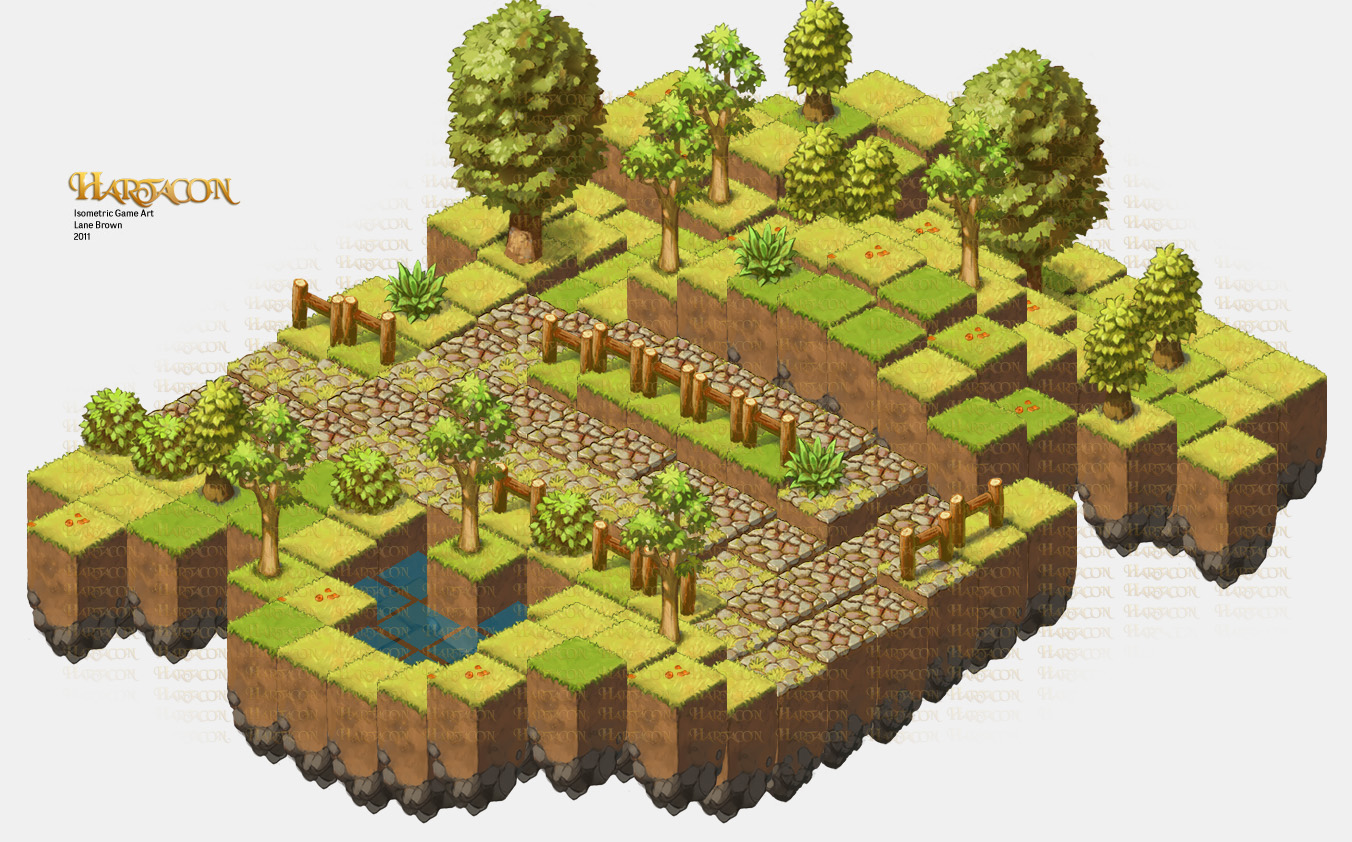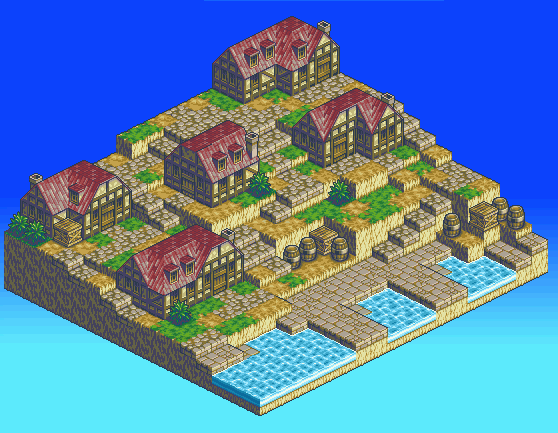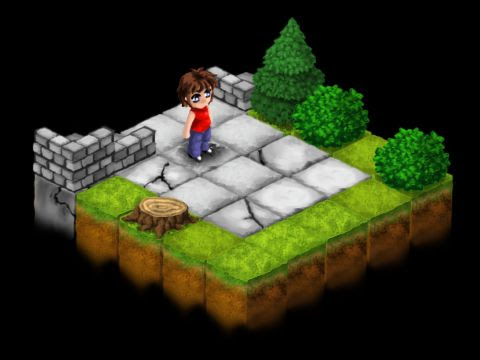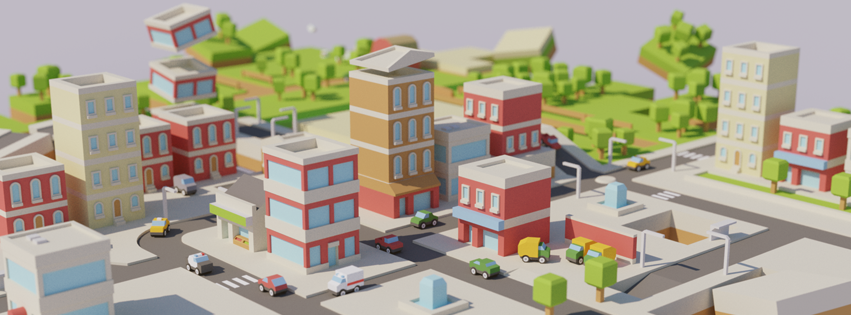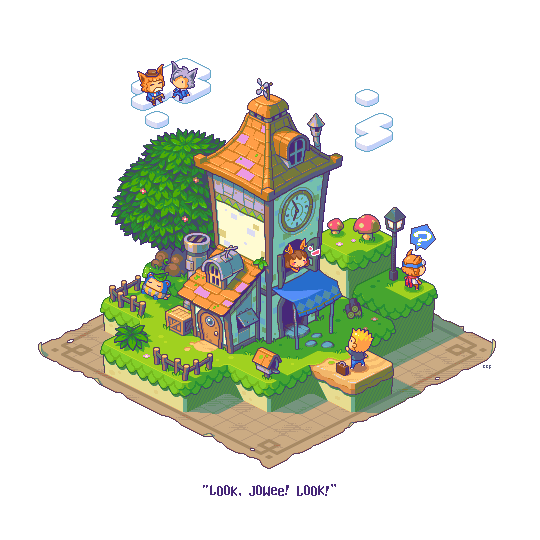## Tuesday, June 30, 2015

### Approximate functions.

For game development, I often use function from the math library, as there are: sinf(), cosf(), atan2f(), acosf(), logf() and such. And these functions tend to be quite expensive in CPU cycles. However, in game development, low precision math functions will often suffice. Which means we can skip computing a precise answer, and use other functions that approximate the output of the expensive functions.

I've found a few resources for code that approximates expensive math functions with much cheaper approximations. There is SIMD Math prims by jhjourdan e.g. that comes with approximations for expf(), logf(), cosf() and sinf(). As a bonus, these simpler functions are also easier to vectorize.

But my favourite approximation is that of atan2f(), because I use it so often to convert direction vectors to angles. Here are Volkan Salma's approximations for the atan2f() function.

## Monday, June 22, 2015

### Tile sets

My next game could well be a 2D tile based game. This is mainly, because I want to use my 2D grid based crowd simulation code. So here I will collect some inspiring art work I found with Google. Note: none of the images are mine, they are all copyrighted by their respective authors. I'm just scrap-booking here to see what art work is out there.

An interesting variant of tiles on a 2D grid, is the use of a height-map, so that there are still NxM tiles on a grid, but each tile has a height. The advantage of doing this over free 3D, is that the navigation on a heightmap is still a 2D affair.